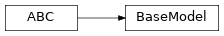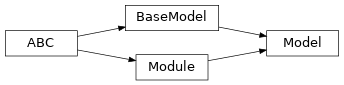# garage.tf.models.model¶

Base model classes.

class BaseModel

Bases: abc.ABCInterface-only abstract class for models.

A Model contains the structure/configuration of a set of computation graphs, or can be understood as a set of networks. Using a model requires calling build() with given input placeholder, which can be either tf.compat.v1.placeholder, or the output from another model. This makes composition of complex models with simple models much easier.

Examples

model = SimpleModel(output_dim=2) # To use a model, first create a placeholder. # In the case of TensorFlow, we create a tf.compat.v1.placeholder. input_ph = tf.compat.v1.placeholder(tf.float32, shape=(None, 2))

# Building the model output = model.build(input_ph)

# We can also pass the output of a model to another model. # Here we pass the output from the above SimpleModel object. model_2 = ComplexModel(output_dim=2) output_2 = model_2.build(output)

build(self, *inputs, name=None)

Output of model with the given input placeholder(s).

This function is implemented by subclasses to create their computation graphs, which will be managed by Model. Generally, subclasses should implement build() directly.

Parameters
• inputs (object) – Input(s) for the model.

• name (str) – Name of the model.

Returns

Output(s) of the model.

Return type

list[tf.Tensor]

property name(self)

Name for this Model.

property parameters(self)

Parameters of the Model.

The output of a model is determined by its parameter. It could be the weights of a neural network model or parameters of a loss function model.

Returns

Parameters.

Return type

list[tf.Tensor]

class Network

Network class For TensorFlow.

A Network contains connectivity information by inputs/outputs. When a Network is built, it appears as a subgraph in the computation graphs, scoped by the Network name. All Networks built with the same model share the same parameters, i.e same inputs yield to same outputs.

property input(self)

Tensor input of the Network.

Returns

Input.

Return type

tf.Tensor

property inputs(self)

Tensor inputs of the Network.

Returns

Inputs.

Return type

list[tf.Tensor]

property output(self)

Tensor output of the Network.

Returns

Output.

Return type

tf.Tensor

property outputs(self)

Tensor outputs of the Network.

Returns

Outputs.

Return type

list[tf.Tensor]

class Model(name)Model class for TensorFlow.

A TfModel only contains the structure/configuration of the underlying computation graphs. Connectivity information are all in Network class. A TfModel contains zero or more Network.

When a Network is created, it reuses the parameter from the model. If a Network is built without given a name, the name “default” will be used.

* Do not call tf.global_variable_initializers() after building a model as it will reassign random weights to the model. The parameters inside a model will be initialized when calling build(). *

Pickling is handled automatcailly. The target weights should be assigned to self._default_parameters before pickling, so that the newly created model can check if target weights exist or not. When unpickled, the unserialized model will load the weights from self._default_parameters.

The design is illustrated as the following:

input_1 input_2

============== Model (TfModel)=================== | | | | | | Parameters | | | ============= / ============ | | | default | / | Network2 | | | | (Network) |/ |(Network) | | | ============= ============ | | | | | =================================================

(outputs from ‘default’ networks) |

outputs from [‘Network2’] network

Examples are also available in tests/garage/tf/models/test_model.

Parameters

name (str) – Name of the model. It will also become the variable scope of the model. Every model should have a unique name.

build(self, *inputs, name=None)

Build a Network with the given input(s).

* Do not call tf.global_variable_initializers() after building a model as it will reassign random weights to the model. The parameters inside a model will be initialized when calling build(). *

It uses the same, fixed variable scope for all Networks, to ensure parameter sharing. Different Networks must have an unique name.

Parameters
• inputs (list[tf.Tensor]) – Tensor input(s), recommended to be positional arguments, for example, def build(self, state_input, action_input, name=None).

• name (str) – Name of the model, which is also the name scope of the model.

Raises

ValueError – When a Network with the same name is already built.

Returns

Output tensors of the model with the given

inputs.

Return type

list[tf.Tensor]

network_input_spec(self)

Network input spec.

Returns

List of key(str) for the network inputs.

Return type

list[str]

network_output_spec(self)

Network output spec.

Returns

List of key(str) for the network outputs.

Return type

list[str]

property parameters(self)

Parameters of the model.

Returns

Parameters

Return type

np.ndarray

property name(self)

Name (str) of the model.

This is also the variable scope of the model.

Returns

Name of the model.

Return type

str

property input(self)

Default input of the model.

When the model is built the first time, by default it creates the ‘default’ network. This property creates a reference to the input of the network.

Returns

Default input of the model.

Return type

tf.Tensor

property output(self)

Default output of the model.

When the model is built the first time, by default it creates the ‘default’ network. This property creates a reference to the output of the network.

Returns

Default output of the model.

Return type

tf.Tensor

property inputs(self)

Default inputs of the model.

When the model is built the first time, by default it creates the ‘default’ network. This property creates a reference to the inputs of the network.

Returns

Default inputs of the model.

Return type

list[tf.Tensor]

property outputs(self)

Default outputs of the model.

When the model is built the first time, by default it creates the ‘default’ network. This property creates a reference to the outputs of the network.

Returns

Default outputs of the model.

Return type

list[tf.Tensor]

reset(self, do_resets=None)

Reset the module.

This is effective only to recurrent modules. do_resets is effective only to vectoried modules.

For a vectorized modules, do_resets is an array of boolean indicating which internal states to be reset. The length of do_resets should be equal to the length of inputs.

Parameters

do_resets (numpy.ndarray) – Bool array indicating which states to be reset.

property state_info_specs(self)

State info specification.

Returns

keys and shapes for the information related to the

module’s state when taking an action.

Return type

List[str]

property state_info_keys(self)

State info keys.

Returns

keys for the information related to the module’s state

when taking an input.

Return type

List[str]

terminate(self)

Clean up operation.

get_trainable_vars(self)

Get trainable variables.

Returns

A list of trainable variables in the current

variable scope.

Return type

List[tf.Variable]

get_global_vars(self)

Get global variables.

Returns

A list of global variables in the current

variable scope.

Return type

List[tf.Variable]

get_regularizable_vars(self)

Get all network weight variables in the current scope.

Returns

A list of network weight variables in the

current variable scope.

Return type

List[tf.Variable]

get_params(self)

Get the trainable variables.

Returns

A list of trainable variables in the current

variable scope.

Return type

List[tf.Variable]

get_param_shapes(self)

Get parameter shapes.

Returns

A list of variable shapes.

Return type

List[tuple]

get_param_values(self)

Get param values.

Returns

Values of the parameters evaluated in

the current session

Return type

np.ndarray

set_param_values(self, param_values)

Set param values.

Parameters

param_values (np.ndarray) – A numpy array of parameter values.

flat_to_params(self, flattened_params)

Unflatten tensors according to their respective shapes.

Parameters

flattened_params (np.ndarray) – A numpy array of flattened params.

Returns

A list of parameters reshaped to the

shapes specified.

Return type

List[np.ndarray]